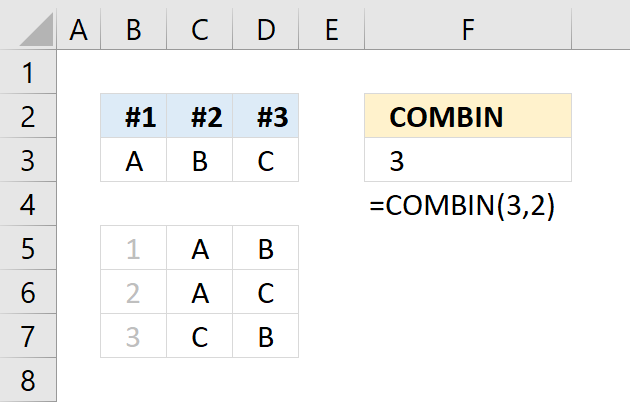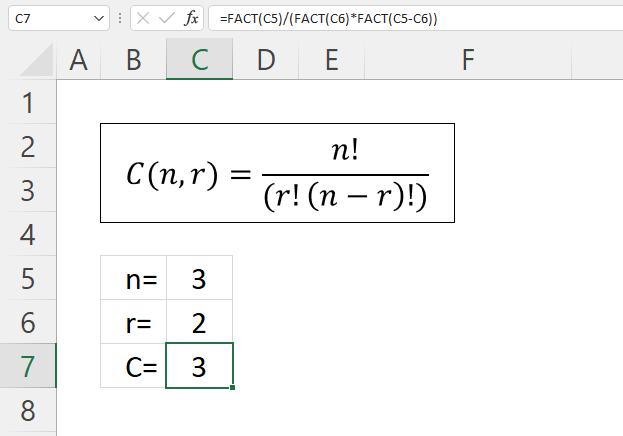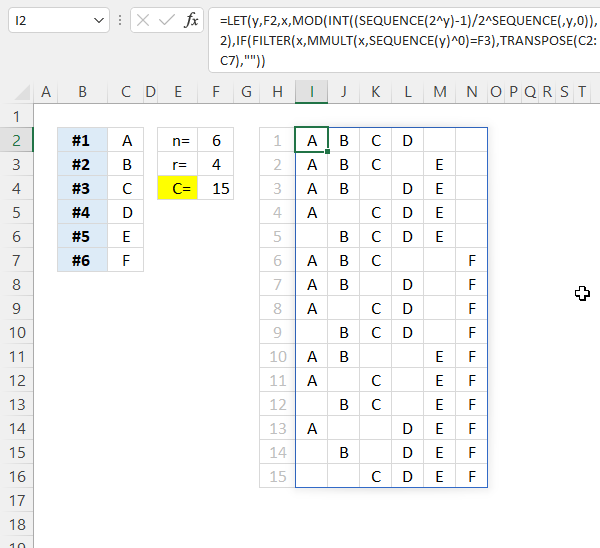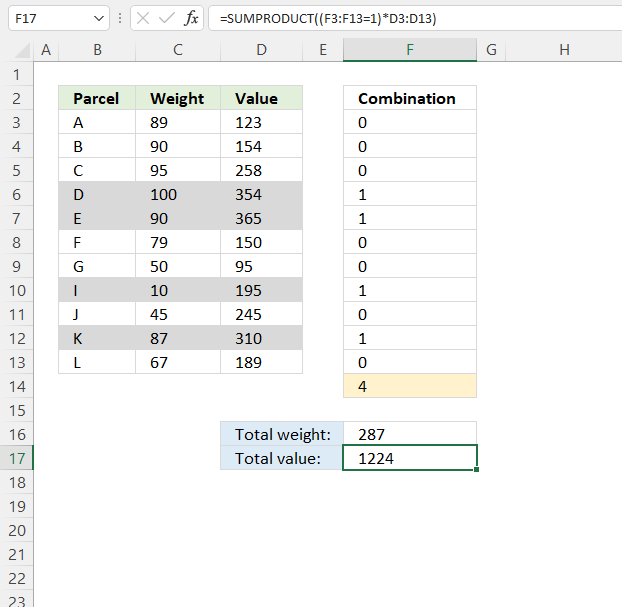Author: Oscar Cronquist Article last updated on May 18, 2022The COMBIN function returns the number of combinations for a specific number of elements out of a larger number of elements.

## 1. COMBIN Function Syntax

COMBIN(number, number_chosen)

## 2. COMBIN Function Arguments

 number Required. A whole number larger than 0 (zero) represents the total number of elements. number_chosen Required. A whole number larger than 0 (zero) represents the number of elements in each combination.

I recommend you press with left mouse button on the following link if you want to read about the difference between combinations and permutations in greater detail.

## 3. COMBIN Function ExampleFormula in cell F3:

=COMBIN(3,2)

Column B, C and D demonstrate how many combinations there are when 2 elements are selected out of 3 elements [A, B, C].

The three combinations are [A,B] ,[A,C] and [B,C]. The elements' internal order is not important, that is why [A,B] and [B,A] is the same combination.

## 4. COMBIN Function alternativeHere is how the COMBIN function calculates in greater detail, see the image above. The formula below is the same as the formula shown in the image above, FACT function is the ! (factorial character).
C = Combinations
n = objects (number)
r = sample (number_chosen)

Formula in cell C7:

=FACT(C5)/(FACT(C6)*FACT(C5-C6))

### 3.1 Explaining formula

#### Step 1 - Calculate the numerator

The FACT function calculates the factorial of a number.

FACT(number)

FACT(C5)

becomes

FACT(3)

and returns 6. 3*2*1 equals 6.

#### Step 2 - Calculate the denominator

(FACT(C6)*FACT(C5-C6))

C5-C6

becomes

3-2 and returns 1.

FACT(1) is 1.

FACT(2) is 2. 2*1 equals 2.

The parentheses let you control the order of operation.

(FACT(C6)*FACT(C5-C6))

becomes

2*1 equals 2.

#### Step 3 - Calculate the division

FACT(C5)/(FACT(C6)*FACT(C5-C6))

becomes

6/2 equals 3.

## 5. Create combinations - Excel 365 formulaExcel 365 dynamic array formula in cell I2:

=LET(y, F2, x, MOD(INT((SEQUENCE(2^y)-1)/2^SEQUENCE(, y, 0)), 2), IF(FILTER(x, MMULT(x, SEQUENCE(y)^0)=F3), TRANSPOSE(C2:C7), ""))

### 4.1 Explaining formula

#### Step 1 - To the power of

This step calculates the number of rows needed to calculate every combination.

2^F2

becomes

2^6 equals 64.

#### Step 2 - Create an array from 0 (zero) to n

The SEQUENCE function creates a sequence of numbers.

SEQUENCE(rows, [columns], [start], [step])

SEQUENCE(2^F2)-1

becomes

SEQUENCE(64)-1

becomes

{1; 2; 3; ... ; 64} - 1

and returns

{0; 1; 2; 3; ... ; 63}.

#### Step 3 -

(SEQUENCE(2^F2)-1)/2^SEQUENCE(,F2,0)

becomes

{0; 1; 2; 3; ... ; 63}/2^SEQUENCE(,F2,0)

becomes

{0; 1; 2; 3; ... ; 63}/2^{0,1,2,3,4,5}

becomes

{0; 1; 2; 3; ... ; 63}/{1,2,4,8,16,32}

and returns

{0, 0, 0, 0, 0, 0;1,  ... , 1.96875}.

#### Step 3 - Remove decimals

The INT function removes the decimal part from positive numbers and returns the whole number (integer) except negative values are rounded down to the nearest integer.

INT(number)

INT((SEQUENCE(2^F2)-1)/2^SEQUENCE(,F2,0))

becomes

INT({0, 0, 0, 0, 0, 0;1,  ... , 1.96875})

and returns

{0, 0, 0, 0, 0, 0; 1, ... , 1}

#### Step 4 - Calculate the remainder

The MOD function returns the remainder after a number is divided by a divisor.

MOD(numberdivisor)

MOD(INT((SEQUENCE(2^F2)-1)/2^SEQUENCE(,F2,0)),2)

becomes

MOD({0, 0, 0, 0, 0, 0; 1, ... , 1}, 2)

and returns

{0, 0, 0, 0, 0, 0; 1, ... , 1}.

#### Step 5 - Calculate the matrix product of two arrays

The MMULT function calculates the matrix product of two arrays, an array as the same number of rows as array1 and columns as array2.

MMULT(array1, array2)

MMULT(MOD(INT((SEQUENCE(2^F2)-1)/2^SEQUENCE(, F2,0)), 2), SEQUENCE(F2)^0)

becomes

MMULT({0, 0, 0, 0, 0, 0; 1,  ... , 1}, {1; 1; 1; 1; 1; 1})

and returns

{0; 1; 1; 2; 1;  ... ; 6}

#### Step 6 - Check if number is equal to numbers_chosen

The equal sign lets you compare value to value in an Excel formula, the result is a boolean value TRUE or FALSE.

MMULT(MOD(INT((SEQUENCE(2^F2)-1)/2^SEQUENCE(,F2,0)),2),SEQUENCE(F2)^0)=F3

becomes

{0; 1; 1; 2; 1;  ... ; 6}=4

and returns

{FALSE; FALSE; FALSE; FALSE;  ... ; FALSE}

#### Step 7 - Filter combinations based on the number chosen

The FILTER function lets you extract values/rows based on a condition or criteria.

FILTER(arrayinclude, [if_empty])

FILTER(MOD(INT((SEQUENCE(2^F2)-1)/2^SEQUENCE(,F2,0)),2),MMULT(MOD(INT((SEQUENCE(2^F2)-1)/2^SEQUENCE(,F2,0)),2),SEQUENCE(F2)^0)=F3)

becomes

FILTER(MOD(INT((SEQUENCE(2^F2)-1)/2^SEQUENCE(,F2,0)),2),{FALSE; FALSE; FALSE; FALSE;  ... ; FALSE})

becomes

FILTER({0, 0, 0, 0, 0, 0; 1, ... , 1},{FALSE; FALSE; FALSE; FALSE;  ... ; FALSE})

and returns

{1, 1, 1, 1, 0, 0;
1, 1, 1, 0, 1, 0;
1, 1, 0, 1, 1, 0;
1, 0, 1, 1, 1, 0;
0, 1, 1, 1, 1, 0;
1, 1, 1, 0, 0, 1;
1, 1, 0, 1, 0, 1;
1, 0, 1, 1, 0, 1;
0, 1, 1, 1, 0, 1;
1, 1, 0, 0, 1, 1;
1, 0, 1, 0, 1, 1;
0, 1, 1, 0, 1, 1;
1, 0, 0, 1, 1, 1;
0, 1, 0, 1, 1, 1;
0, 0, 1, 1, 1, 1}

#### Step 8 - Populate array

The IF function returns one value if the logical test is TRUE and another value if the logical test is FALSE.

IF(logical_test, [value_if_true], [value_if_false])

IF(FILTER(MOD(INT((SEQUENCE(2^F2)-1)/2^SEQUENCE(,F2,0)),2),MMULT(MOD(INT((SEQUENCE(2^F2)-1)/2^SEQUENCE(,F2,0)),2),SEQUENCE(F2)^0)=F3),TRANSPOSE(C2:C7),"")

becomes

IF({1, 1, 1, 1, 0, 0;
1, 1, 1, 0, 1, 0;
1, 1, 0, 1, 1, 0;
1, 0, 1, 1, 1, 0;
0, 1, 1, 1, 1, 0;
1, 1, 1, 0, 0, 1;
1, 1, 0, 1, 0, 1;
1, 0, 1, 1, 0, 1;
0, 1, 1, 1, 0, 1;
1, 1, 0, 0, 1, 1;
1, 0, 1, 0, 1, 1;
0, 1, 1, 0, 1, 1;
1, 0, 0, 1, 1, 1;
0, 1, 0, 1, 1, 1;
0, 0, 1, 1, 1, 1},TRANSPOSE(C2:C7),"")

The TRANSPOSE function allows you to convert a vertical range to a horizontal range, or vice versa.

TRANSPOSE(array)

TRANSPOSE(C2:C7)

becomes

TRANSPOSE({"A"; "B"; "C"; "D"; "E"; "F"})

and returns

{"A", "B", "C", "D", "E", "F"}.

IF({1, 1, 1, 1, 0, 0;
...
0, 0, 1, 1, 1, 1},TRANSPOSE(C2:C7),"")

becomes

IF({1, 1, 1, 1, 0, 0;
...
0, 0, 1, 1, 1, 1},{"A", "B", "C", "D", "E", "F"},"")

and returns

{"A", "B", "C", "D", "", "";
"A", "B", "C", "", "E", "";
"A", "B", "", "D", "E", "";
"A", "", "C", "D", "E", "";
"", "B", "C", "D", "E", "";
"A", "B", "C", "", "", "F";
"A", "B", "", "D", "", "F";
"A", "", "C", "D", "", "F";
"", "B", "C", "D", "", "F";
"A", "B", "", "", "E", "F";
"A", "", "C", "", "E", "F";
"", "B", "C", "", "E", "F";
"A", "", "", "D", "E", "F";
"", "B", "", "D", "E", "F";
"", "", "C", "D", "E", "F"}

#### Step 9 - Simplify formula

The LET function lets you  name intermediate calculation results which can shorten formulas considerably and improve performance.

LET(name1name_value1calculation_or_name2,
[name_value2calculation_or_name3...])

IF(FILTER(MOD(INT((SEQUENCE(2^F2)-1)/2^SEQUENCE(,F2,0)),2),MMULT(MOD(INT((SEQUENCE(2^F2)-1)/2^SEQUENCE(,F2,0)),2),SEQUENCE(F2)^0)=F3),TRANSPOSE(C2:C7),"")

x - MOD(INT((SEQUENCE(2^y)-1)/2^SEQUENCE(, y, 0)), 2)
y - F2

LET(y, F2, x, MOD(INT((SEQUENCE(2^y)-1)/2^SEQUENCE(, y, 0)), 2), IF(FILTER(x, MMULT(x, SEQUENCE(y)^0)=F3), TRANSPOSE(C2:C7), ""))

## 6. Test combinations (Solver)The Solver is an Excel feature that can test combinations in order to find the best combination based on a condition or criteria. This example shows parcel names in column B, weight in column B and the value in column D.

Which combination of four parcels out of 11 have the highest value if the total weight is lower than or equal to 291?

Total weight formula in cell F16:

=SUMPRODUCT((F3:F13=1)*C3:C13)

Total value formula in cell F17:

=SUMPRODUCT((F3:F13=1)*D3:D13)

### Explaining the total weight formula

#### Step 1 - Compare values

The equal sign is a logical operator that lets you check if a value is equal to another value, in this case, multiple values to a single value.

The Excel Solver changes the numbers, 1 or 0 (zero), in cells F3:F13. We use these values to calculate the total weight for each combination.

F3:F13=1

(F3:F13=1)

#### Step 3 -

(F3:F13=1)*C3:C13

#### Step 4 -

SUMPRODUCT((F3:F13=1)*C3:C13)